Math Calculators, Lessons and Formulas

It is time to solve your math problem

mathportal.org
• Geometry
• Triangular pyramid

# Triangular pyramid

ans:
syntax error
C
DEL
ANS
±
(
)
÷
×
7
8
9
4
5
6
+
1
2
3
=
0
.
auto next question
calculator
•  Question 1: 1 pts The surface area of the regular triangular pyramid shown on the picture is $A=\left(\dfrac{5^{2}\sqrt{3}}{4}+3\cdot\dfrac{5\cdot 10}{2}\right)cm^{2}$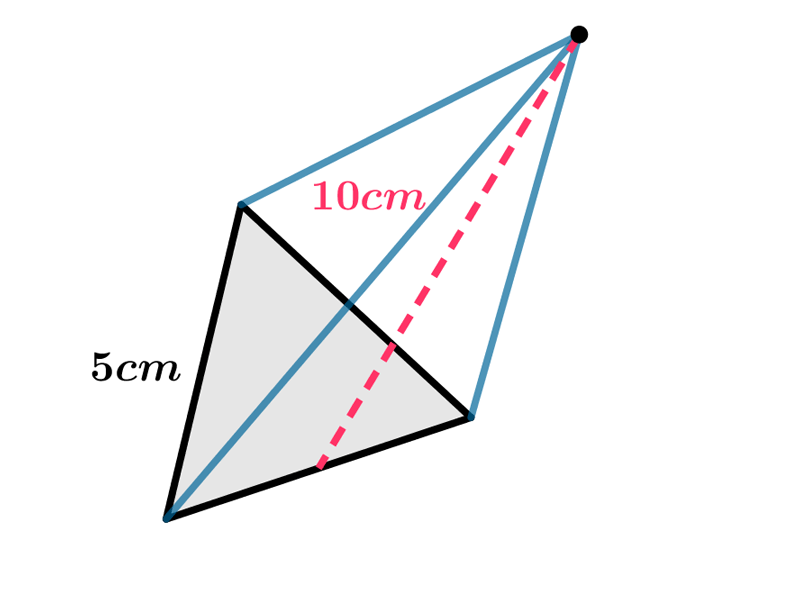•  Question 2: 1 pts The following expression can be used to find the height of the regular triangular pyramid shown on the picture.$$9^{2}=H^{2}+(\sqrt{3})^{2}$$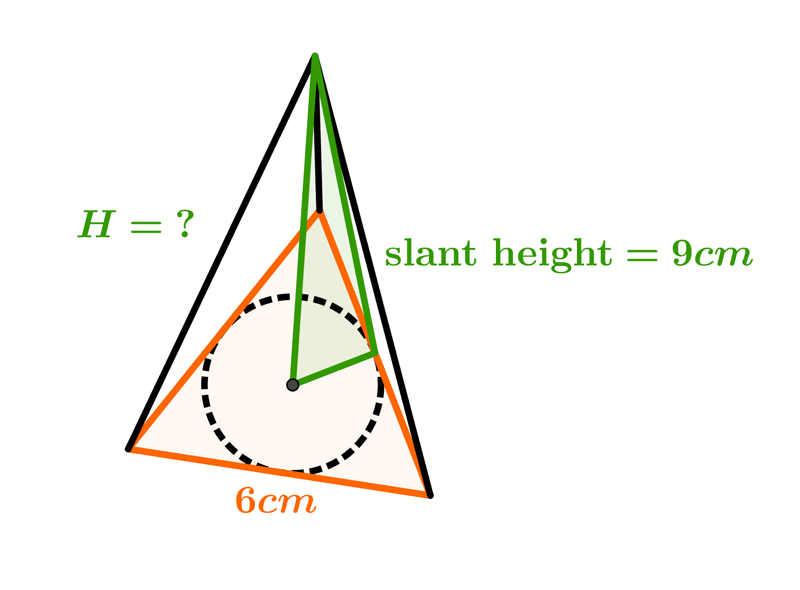•  Question 3: 1 pts The following expression can be used to find the volume of the regular triangular pyramid shown on the picture. $$V=\left(\dfrac{\left(6\sqrt{3}\right)^{2}\sqrt{3}}{4}\cdot 4 \right)cm^{3}$$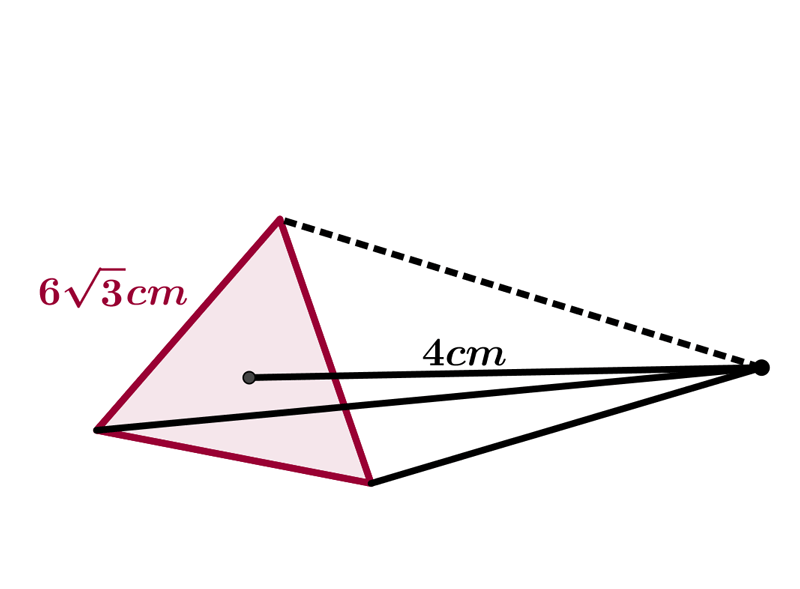•  Question 4: 1 pts The following expression can be used to find the length of base edges of the regular triangular pyramid shown on the picture. $8^{2}=4^{2}+x^{2}$ $\mbox{base edge}=\dfrac{6x}{\sqrt{3}}$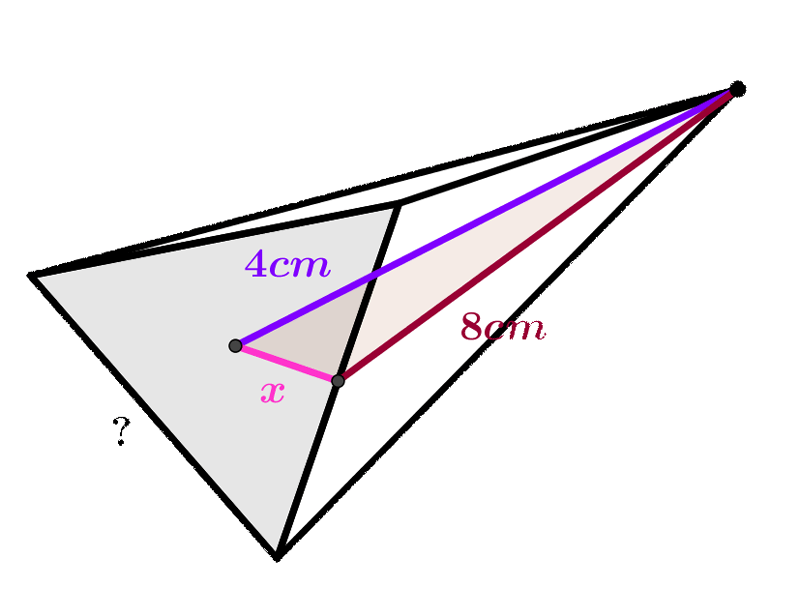•  Question 5: 2 pts Determine the volume and surface area of a regular triangular pyramid having a base edge $a=10 cm$ and a lateral edge $b = 13 cm.$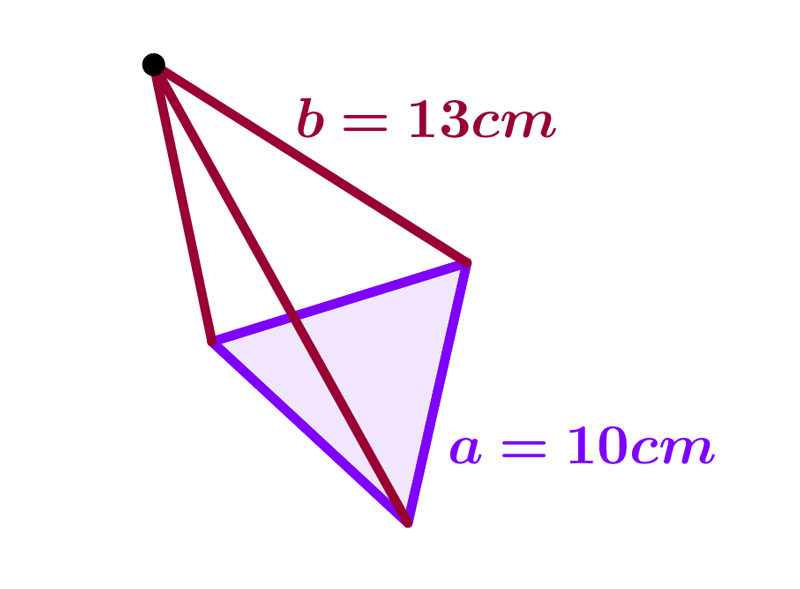•  Question 6: 2 pts Calculate the volume of a regular triangular pyramid whose height is equal to the length of the base edges $11 cm.$
 $V=\dfrac{1331\sqrt{3}}{12}cm^{3}$ $V=\dfrac{985\sqrt{3}}{12}cm^{3}$ $V=\dfrac{613\sqrt{3}}{8}cm^{3}$ $V=\dfrac{613\sqrt{3}}{4}cm^{3}$
•  Question 7: 2 pts �Area of the whole surface of the regular tetrahedron $$A=a^{2}\sqrt{3}$$
•  Question 8: 2 pts Volume of the regular tetrahedron $$V=\dfrac{a^{3}\sqrt{2}}{\sqrt{3}}$$
•  Question 9: 3 pts Which expression can be used to find the height of regular triangular pyramid if her surface area is $112\sqrt{3}cm^{2},$ and the length of base edge is $8cm.$
 $H^{2}=\left(4\sqrt{3}\right)^{2}+\left(\dfrac{4\sqrt{3}}{3}\right)^{2}$ $H^{2}=\left(8\sqrt{3}\right)^{2}-\left(\dfrac{4\sqrt{3}}{3}\right)^{2}$ $H^{2}=\left(6\sqrt{3}\right)^{2}+\left(\dfrac{4\sqrt{3}}{3}\right)^{2}$ $H^{2}=\left(2\sqrt{3}\right)^{2}-\left(\dfrac{3\sqrt{3}}{4}\right)^{2}$
•  Question 10: 3 pts The area of total lateral surface of the triangular pyramid is $162 cm^{2}.$ Find the length of base edge of that pyramid if the base edge is equal to apothem.
 $4\sqrt{5}cm$ $6\sqrt{3}cm$ $4\sqrt{2}cm$ $3\sqrt{6}cm$
•  Question 11: 3 pts Find the volume of the triangular pyramid shown on the picture .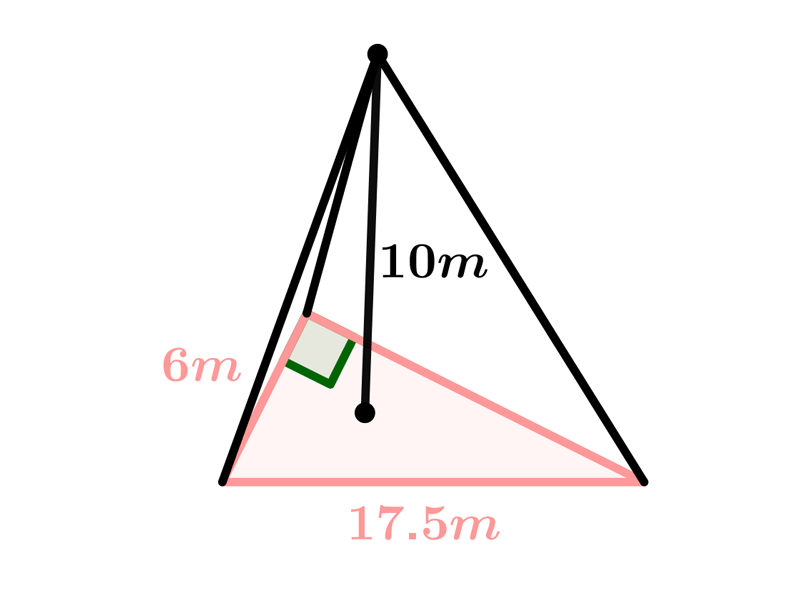$V=175\sqrt{3}m^{3}$ $V=196\sqrt{3}m^{3}$ $V=221\sqrt{3}m^{3}$ $V=343\sqrt{3}m^{3}$
•  Question 12: 3 pts Find the volume of the triangular pyramid shown on the picture .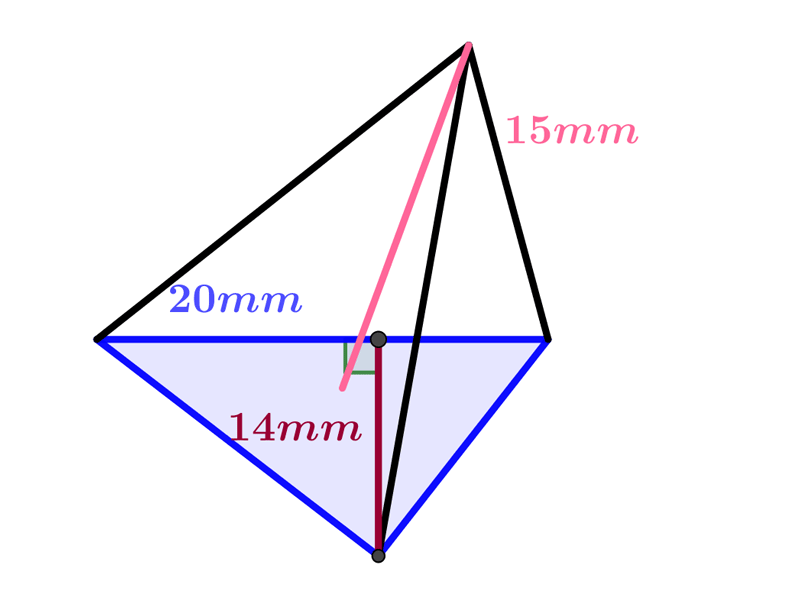$V=545\sqrt{3}m^{3}$ $V=235\sqrt{3}m^{3}$ $V=425\sqrt{3}m^{3}$ $V=700\sqrt{3}m^{3}$